### Unity Voxel Tutorial Part 4: Destroying and placing blocks

#### Hello everyone following the voxel tutorial, it's been a long time since an update. In this time I've written a new updated tutorial on voxel terrain that supports infinite terrain and saving/loading of chunks. Try it out on my new site: AlexStv.com

Now that we have semi realistic terrain we need to be able to manipulate the terrain in real time because that's kind of a staple of these kinds of games or even the point of these kinds of games. The difficulty of this mostly comes down to converting floating point positions from unity to coordinates in our grid array. Our grid array is offset by a little so we need to figure out these offsets. We could also adjust our block generation to align it better to the real positions but this solution is easier.

What we'll start off with is a raycasting script to destroy blocks. Make a new script and call it "RaycastExample". Give it the following variables:
```public GameObject terrain;
private PolygonGenerator tScript;
public GameObject target;
```

The terrain GameObject will refer to the object with the "PolygonGenerator" script and in the start function we'll get the polygon generator script and save it as the tScript variable:

```void Start () {
tScript=terrain.GetComponent("PolygonGenerator") as PolygonGenerator;
}
```

Now in the update function we'll raycast every frame from this object to the target object destroying the first block hit.
```void Update () {

RaycastHit hit;

float distance=Vector3.Distance(transform.position,target.transform.position);

if( Physics.Raycast(transform.position, (target.transform.position -
transform.position).normalized, out hit, distance , layerMask)){

Debug.DrawLine(transform.position,hit.point,Color.red);

} else {
Debug.DrawLine(transform.position,target.transform.position,Color.blue);
}
}
```

If you haven't used raycasts before I won't go into the basics here but essentially it's the origin of the ray, the direction that we calculate using the origin and the target location, the variable we'll output the hit data to, the max distance of the ray which is calculated earlier as the distance between origin and target, and lastly the layer mask which is which layers this ray collides with. The layermask we've already defined in the start, it's set to 0 which is the default layer. This way you could have characters or entities on another layer and not have them stop the raycasts.

Add the script to a gameobject and create another gameobject to set as the target, make sure they are both at 10 z. Also set the raycaster's terrain variable to the gameobject containing the polygon generator.

If you run it now you should see a line drawn in red to the hit point if it collides and a blue line to the target if not.The raycast hitting the terrain.
Now we'll use the location of the hit to figure out which block it is. So create a vector2 with the hit.point's x and y and then add the inverse of the hit's x and y normals. What this does is that it gives use the hit location and then moves it further into the block using the normals.
``` if( Physics.Raycast(transform.position, (target.transform.position -
transform.position).normalized, out hit, distance , layerMask)){

Debug.DrawLine(transform.position,hit.point,Color.red);

Vector2 point= new Vector2(hit.point.x, hit.point.y);   //Add this line
point+=(new Vector2(hit.normal.x,hit.normal.y))*-0.5f;  //And this line

} else {
Debug.DrawLine(transform.position,target.transform.position,Color.blue);
}
}```

What these lines do is that they take the position of the collision (hit.point) and create a new vector2 with the position, then we add to that Vector2 half of the reverse of the normal of the surface hit. The normal is the direction that would point straight away from the surface, so adding the reverse takes us 1 unit further into the block but half that takes us half a unit further in where we can round to the position of the block.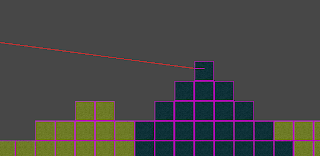You won't see this but this is a visualization of the raycast to the hitpoint and then a line to the new point with the inverse normals added, you can see that it now ends up inside the block.
Now we'll set the block at this point to air:
```tScript.blocks[Mathf.RoundToInt(point.x-.5f),Mathf.RoundToInt(point.y+.5f)]=0;
```

This goes after the vector2 point is defined and adjusted. It rounds the point's x and y to ints to that we can use them to choose points in the array. First though you have to subtract .5f from x and add .5f from y because of the terrain's offset from the world coordinates. You wouldn't need this if block 0,0's center was at 0,0 but it isn't.

Now to update the blocks but instead of just rebuilding and updating the mesh remotely we'll use the polygon generator's update to let it do it. Go back to the polygon generator and add a public bool called update.

```public bool update=false;
```

Then create an Update function for the polygon generator. In the Update loop add this:
```void Update(){
if(update){
BuildMesh();
UpdateMesh();
update=false;
}
}
```

This way we can set update to true remotely and the mesh will update but the best part is that even if several scripts change blocks they will and just change update to true but the mesh will only update once for all of them when its Update loop runs.

So set update to true for our polygon generator from the raycast script:
```tScript.update=true;
```The raycast should destroy one block per frame until it reaches it's target.
Sweet, now we have lasers. You could easily convert this to a part of a player script to run once and destry the block for example in front of the player.

Now that's not all though, sometimes you don't want to destroy a block at a specific point, you want to destroy all the blocks in a general area. For this we'll make a new script "ColliderExample". Give it the following variables:
```public GameObject terrain;
private PolygonGenerator tScript;
public int size=4;
public bool circular=false;
```

And we'll use the same start code to get the Polygon Generator script
```void Start () {
tScript=terrain.GetComponent("PolygonGenerator") as PolygonGenerator;
}
```
Because this is going to be removing a lot of blocks at once we'll make a RemoveBlock function:

```bool RemoveBlock(float offsetX, float offsetY){
int x =Mathf.RoundToInt(transform.position.x+offsetX);
int y=Mathf.RoundToInt(transform.position.y+1f+offsetY);

if(x<tScript.blocks.GetLength(0) && y<tScript.blocks.GetLength(1) && x>=0 && y>=0){

if(tScript.blocks[x,y]!=0){
tScript.blocks[x,y]=0;
return true;
}
}
return false;
}
```

What we do here is very similar to the last remove block code we wrote only this time we also check first to see if the block is within the bounds of the array (in case our object is placed close to the edge). Then if the block isn't already air we just set the block to zero and return true to let the script that calls it know that a change was made.

Now in the update loop we'll run the remove block script for all the blocks in an area. I'll just paste in the whole chunk:
```void Update () {

bool collision=false;
for(int x=0;x<size;x++){
for(int y=0;y<size;y++){
if(circular){

if(Vector2.Distance(new Vector2(x-(size/2),
y-(size/2)),Vector2.zero)<=(size/3)){

if(RemoveBlock(x-(size/2),y-(size/2))){

collision=true;
}

}
} else {

if(RemoveBlock(x-(size/2),y-(size/2))){

collision=true;
}
}

}
}
if( collision){
tScript.update=true;

}

}
```

So we run this for each block in a square of size by size, if the circular bool is true then first we check to see if the distance from the origin is smaller than one third the size to create a circular effect. Originally I used half the size consistent with everything else but I found that at low values some sides would be cut off so when set to circular the blast radius is smaller that otherwise. Then for both the circular and noncircular parts we remove the block at that point subtracting half the size (Because otherwise the object would be in the top left of the blast, this offsets it to the center) if the remove block function returns true we set the collision we defined earlier to true. After the loops if anything set collision to true we update the polygon generator, this way we don't update the mesh if nothing was removed.Apply the script to a gameobject and you can do this.
Now you have both area and specific point block removers. You could even combine them and do this: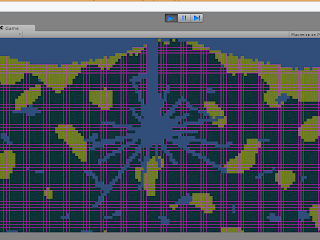For explosive effects
Now I also promised creating blocks, this will work exactly like the raycast code. Make a new script called BlockPlaceExample and copy the contents of the raycast example there but replace the name with BlockPlaceExample.

Now all you have to change is instead of multiplying the normals that you add to the hit point by -0.5f you just multiply by 0.5f to get the block next to the block hit. And instead of setting it to air you set it to whatever you want. For example:

```point+=(new Vector2(hit.normal.x,hit.normal.y))*0.5f;
```

`tScript.blocks[Mathf.RoundToInt(point.x-.5f),Mathf.RoundToInt(point.y+.5f)]=1;`

And instead of destroying a block every frame you will build one.It looks kind of freaky.
Now instead of running it every frame you could run it once and instead of using a target just shoot it one meter to the left or right of the player and place a block!

And that's part 4, message me with any problems you find or feedback you think of. Follow me on twitter (@STV_Alex) or G+ to get updated when I post part five!

Completed code for the tutorial so far: http://studentgamedev.blogspot.no/p/part-4-complete-code.html

Part 5

1.I'm a little stuck, when I get to this step:

"Now we'll use the location of the hit to figure out which block it is.

Vector2 point= new Vector2(hit.point.x, hit.point.y);
point+=(new Vector2(hit.normal.x,hit.normal.y))*-0.5f;"

where exactly am I adding these lines? Void Start or Update? I might be misreading something, but I can't find anything about where to put it.

Other than that, everything is working fine. Can't wait for part 5! Thanks. :D

1.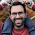Ah that goes just after the Debug.DrawLine inside the Physics.Raycast. I'll update the post to make this more clear, thanks Wolli.

2.Thank you so much for these tutorials, I've found a lot of video tutorials on this subject but I've always been better at learning from text. I'm looking forward to part 5, and when you move into doing this in 3D.

3.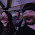would you change the line byte[,] = blocks to byte[,,] = blocks and then go through and add pz through the code to turn it into 3d? I'm still learning how to do this but I actually want to see if I can turn it to 3d with maybe a little input.

Very good tutorial though. This is the first time I've delved into anything like this. Closest I got to actual programming was some minor stuff in C++ and some LUA and excel...not that they are heavy duty coding but at least it gives me a basis for understanding what's being talked about in these tut's.

4.Also ran into a problem trying to set the Raycast's terrain variable to the GameObject with the PolygonGenerator script and it's not listed in the assets. Was I supposed to create a prefab instead?

5.Does anyone else get horrid performance with this? I get 120+ fps until collisions occur, then it drops to seconds per frames. I have had to take down unity with the old three finger salute (CTRL+ALT+Delete)

1.I get a pretty terrible performance hit with RaycastExample and BlockPlaceExample. I normally get around 400+FPS but get dropped down to ~35 FPS. ColliderExample seems to do much better.

2.I know this is an old post, and you probably gave up on it or figured it out yourself, but I had this problem and found the solution. So if anyone is stuck with this problem, this is what caused it for me.

In the PolygonGenerator the mesh.Optimize() was used, but as it has become obsolete I searched for what I could replace it with. I found MeshUtility.Optimize(mesh) and used it. However, this seems to be the cause of the slow-down. So I just removed it.

6.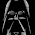Got it. Thats awsome, looks like what they do in terraria. Thanks I am really enjoying your tutorial.

7.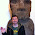Alexandros, nice tutorials. Can't wait to start the 3D ones.

@JohnnySabotage - I'm getting a steady 75fps regardless of using any of block builders, destroyers, or sitting idle.

8.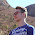Can you please upload the source for the scripts up to the end of this part like you did in the second tutorial?

1.2.9.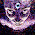This comment has been removed by the author.

10.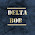Hi,
I don't get how to set up my scene in Unity.
In the beginning with the first picture when we have "The raycast hitting the terrain".
I don't know to what object I need to apply the new script to.

1.You should create a new game object (Ctrl + Shift + N) in the scene to put the script on, this will be the raycast's source. Then also create a gameobject to act as its target and drag the target from the Hierarchy tab to the raycaster's target variable in the Inspector tab. Make sure the z position of both the new gameobjects is 10 so that it's in line with the terrain.

11.I cannot get the raycast hit to register (Unity 4.2.2). The distance displays correctly between the cast and receive object (shows blue) and follows correctly, but I cannot get the ray to intersect the generated poly faces. The object appears to be detected, and it's generator control is being found.

Any ideas? I have been through the code line by line, and will be looking again.

r

1.After doing some digging it looks like the 3d raycasts, as we are using here, don't detect collisions with 2d objects, which is what we have generated.

The best fixes I have found to make this function work is to use
http://docs.unity3d.com/Documentation/ScriptReference/Physics2D.OverlapPoint.html
http://docs.unity3d.com/Documentation/ScriptReference/RaycastHit2D.html

However I will say that getting the line to draw appropriately after detecting from a 3d ray is a pain in the behind. I'd suggest doing a full on conversion to 2dRays and follow the rest of the tutorial normally.

2.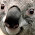The collision mesh is 3d thus its handled as a 3d object.

12.This comment has been removed by the author.

13.This comment has been removed by the author.

14.I don't think this was a mistake on my part cause I tried copied and pasted some of the code. Well here

tScript.blocks[Mathf.RoundToInt(point.x - 0.5f),Mathf.RoundToInt(point.y +0.5f)] = 0;

When I got to this part I got stuck cause nothing would happen when I tried colliding my target with the terrain. I set the byte to = 1 and it would spawn a block on top. Which I found weird because it was supposed to turn the block at the bottom into stone. So after analyzing what was actually happening I tried changing + to - in the y and bam it worked! I don't know if I did something else wrong prior or it was a mistake :\

tScript.blocks[Mathf.RoundToInt(point.x - 0.5f),Mathf.RoundToInt(point.y - 0.5f)] = 0;

15.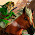As far as I can tell the third screenshot (the laser part) is complete nonsense. The ray never passes thru all of the terrain. It always gets stuck on the corner of a block somewhere. It seems that the problem is the fact that the code does not take into account the orientation of the face it hit. For example if it hits the top-left corner of a block and considers it the top face, it gets the inverse of the normal of that face which moves it down half a block but its still on the left edge of the block at this point. As a result the adjustment moves it one block left, which is not the correct block. So it tries to remove the incorrect block and gets stuck repeatedly colliding with a block it cannot destroy because of this issue with the math.

I cannot seem to get this to work no matter what I do and I'm not sure how the author could miss a problem this serious. I mean, the code simply does not work. I've checked and rechecked it. The suggestion in the above post does not solve it either.

1.Hey guys, I have managed to finally solve this tricky and aggravating problem after hours of experimentation. The result is that I created a function that gets us block coordinates from the triangle that our ray collided with. Basically, it gets the vertices of the triangle the ray collided with. It then tests to see which 2 points on the triangle both reside on the same Z-coordinate as the collision point. If only one point on the triangle has the same Z-coordinate, it duplicates that point to serve as the 2nd point. Using the 2 points we can now find the mid point of the side of the triangle we hit. Then add half of the inverted normal and we get the center point of the block to destroy basically. If there was only one point that got duplicated, this still works because the normal will be at an angle which when inverted will still put the resulting point inside of the block we need to destroy.

This code should work no matter what direction the Raycast comes from as well. Anyway, here is the code of this function for you guys:

2.Vector2 BlockCoordFromHitTriangle(RaycastHit hitInfo, Vector2 rayVector)
{
// Get the collider and its mesh.
MeshCollider meshCollider = hitInfo.collider as MeshCollider;
Mesh mesh = meshCollider.sharedMesh;

// Extract local space coordinates of the triangle that the ray hit
Vector3 p0 = mesh.vertices [mesh.triangles [hitInfo.triangleIndex * 3 + 0]];
Vector3 p1 = mesh.vertices [mesh.triangles [hitInfo.triangleIndex * 3 + 1]];
Vector3 p2 = mesh.vertices [mesh.triangles [hitInfo.triangleIndex * 3 + 2]];

// Transform local coords to world coords.
p0 = hitInfo.collider.transform.TransformPoint(p0);
p1 = hitInfo.collider.transform.TransformPoint(p1);
p2 = hitInfo.collider.transform.TransformPoint(p2);

// Print debug output showing the 3 points of the triangle our ray hit.
print("HIT TRIANGLE");
print("P0: (" + p0.x.ToString() + ", " + p0.y.ToString() + ")");
print("P1: (" + p1.x.ToString() + ", " + p1.y.ToString() + ")");
print("P2: (" + p2.x.ToString() + ", " + p2.y.ToString() + ")");

// This will hold the vertices of the side of the triangle that the ray hit.
Vector2[] sidePoints = new Vector2;

// Detect which two vertices of the triangle belong to the side the ray collided with.
// We do this by finding the two points with the same Z coordinate.
int index = 0;
if ((int)p0.z == (int)hitInfo.point.z)
{
sidePoints [index] = p0;
index++;
}
if ((int)p1.z == (int)hitInfo.point.z)
{
sidePoints [index] = p1;
index++;
}
if ((int)p2.z == (int)hitInfo.point.z)
{
sidePoints [index] = p2;
index++;
}

// If only one point on the triangle is on the same Z coord as the hit point, then simply copy that point into
// the second slot in the sidePoints array. This way the midpoint will be this point itself so the code
// below will still get the correct block coordinates.
if (index < 2)
sidePoints  = sidePoints ;

// Print debug output showing the coordinates of our two points.
print("SideP0: (" + sidePoints .x.ToString() + ", " + sidePoints .y.ToString() + ")");
print("SideP1: (" + sidePoints .x.ToString() + ", " + sidePoints .y.ToString() + ")");

// Find the center point of the side the ray collided with.
Vector2 center = new Vector2((sidePoints .x + sidePoints .x) / 2,
(sidePoints .y + sidePoints .y) / 2);

// Print debug output showing the center point's coordinates.
print("Center Point: (" + center.x.ToString() + ", " + center.y.ToString() + ")");

// Draw the normal for this RaycastHit.
Debug.DrawLine(hitInfo.point, hitInfo.point + hitInfo.normal, Color.cyan);

// Halve and invert the normal.
Vector2 normalInvertedHalved2D = new Vector2(hitInfo.normal.x, hitInfo.normal.y) * -0.5f;

// Add the halved and inverted normal to our center point to get a point inside the block we need to destroy.
return center + normalInvertedHalved2D;
}
}

16.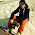Great tutorial, thanks alot. How would you handle player movement with this generated terrain mesh collider?
2D rigidbody? I can't get collisions to work with 2d box collider. Thanks.

17.Hey, I have one question. If I undestood right this polygonGenerator script generate numbers 0, 1, 2 (air, sand, rock) and then turn it into blocks (terrain).

So how can I save that list of numbers. Then maybe change some of those and put it back to the script which will turn it into blocks (terrain). So It wouldn't be "random" generator anymore and I could make different kind of maps with it.

I hope you understood what I mean. Thank you!

1.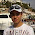I answer to my own question.

I found way to do this by saving "public byte[,] blocks;" data just end of GenTerrain() function.

Then in start() I don't use GenTerrain(), insted I load blocks[,] from memory.

Thats all. Simple as that.

2.Arno. Do you mind sharing your code?

18.This comment has been removed by the author.

19.Still messing around your tutorial ..

if you want to draw the image2 you can add that :
// drawing the position targeted
Vector3 drawpoint = new Vector3(point.x,point.y,hit.point.z); // point is vector2 , we need to add the "Z" dimension
Debug.DrawLine(hit.point,drawpoint,Color.green);

Plus i got an issue , since i used another vector3 to place my GameObject , or the terrain .
To rectify it, I added those vars under tScript declaration :

private PolygonGenerator tScript;
private float tScriptX; // X coordinate of the terrain
private float tScriptY; // Y coordinate of the terrain

And added that when we change the block into air on hit :
tScriptX=tScript.transform.position.x;
tScriptY=tScript.transform.position.y;

tScript.Blocks[Mathf.RoundToInt(point.x-.5f-tScriptX),Mathf.RoundToInt(point.y+.5f-tScriptY)]=0;

this work since we have only one "Z" coordinate for all our objects , and since we work on a 2D example .

20.This comment has been removed by the author.

21.Mathf.floor is well instead of strange rounding.